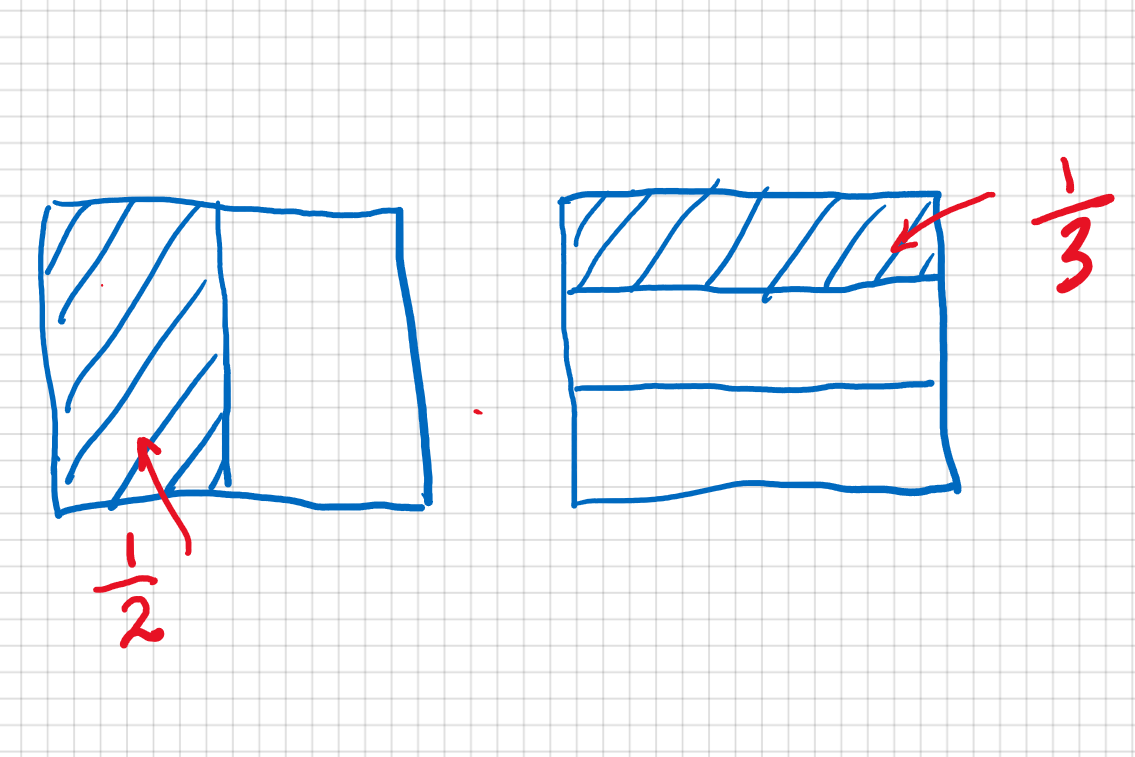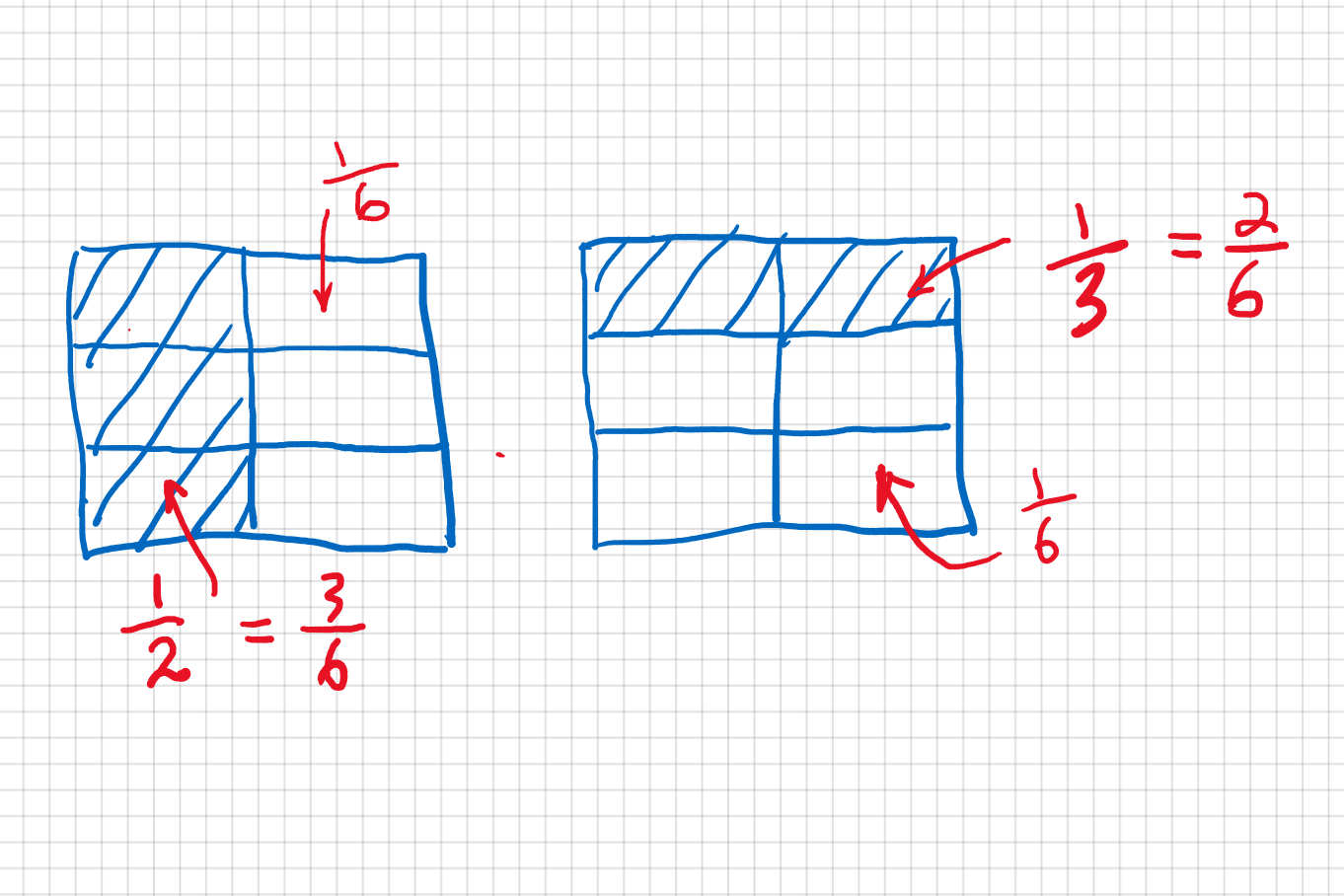# Chapter II: Fractions

Once upon a time, a kindergartener came to me. He was very excited because he just learned addition that day and he wanted to show me. So I asked him what is one apple plus another apple. He answered two apples. Then I asked him what is one banana plus another banana; he said two bananas. Then, I asked him, "what is one apple plus one banana?". He almost said "two", but he paused before making the sound.

You see, he realized that in order to add two quantities, they have to be the same thing. This is in essence the problem with adding fractions because almost all the time, at least in math classes, they have different size. We can not add 1/2 and 1/3 directly because the former is less than the latter.

Let me give you another example. One dollar plus one quarter is not two dollars nor two quarters. We can not add them directly because they have different units. In order to add them, we have to convert one's unit to the other's. For example, we can convert the one dollar to 4 quarters. So one dollar plus one quarter is five quarters. Similarly, we have to do some conversion before we can add fractions.

Let us start by adding 1/2 and 1/3. First, let us understand what they are. That is always a good starting point. The picture below shows what they are.As you can see, 1/2 means cutting a whole thing, whatever it is, into two equal part and each part is called 1/2. Similarly, 1/3 means cutting a whole thing into three equal parts, and each part is called 1/3. And clearly, they have different size. Imagine you have a whole pizza and are very hungry, do you want to share it with one friend or two friends?

What do we do then? The trick is to cut them to smaller pieces like shown in the figure below, so that they have something in common.So now 1/2 is cutting down to three 1/6 and 1/3 to two 1/6. Because they now have a common unit, that is 1/6, we can just add them up to get five 1/6. In mathematics, we'd like to write the whole process as below:

1/2 + 1/3 = 3/6 + 2/6 = 5/6

Now, let us try a couple of more examples, and I am sure you will see a pattern. So let's try adding 1/3 and 1/4.

Here is the formula for simple fraction addition:

1/a + 1/b = (b+a)/(a xx b)

Here a and b are any non-zero integers.

Here are some examples:

1/2 + 1/3 = (3+2)/(2 xx 3) = 5/6
1/7 + 1/5 = (5+7)/(7 xx 5) = 12/35

## B. Simple fraction subraction

Here is the formula for simple fraction subtraction:

1/a - 1/b = (b-a)/(a xx b)

Here a and b are any non-zero integers.

Here are some examples:

1/2 - 1/3 = (3-2)/(2 xx 3) = 1/6
1/5 - 1/7 = (7-5)/(7 xx 5) = 2/35

Here is the formula for fraction addition:

a/b + c/d = (a xx d + b xx c)/(b xx d)

Here a, b, c, and d can be any non-zero integers. a xx d + b xx c is the so-called cross multiplication.

Here are some examples:

2/3 + 4/5 = (2 xx 5 + 3 xx 4)/(3 xx 5) = 22/15
3/7 + 5/6 = (3 xx 6 + 7 xx 5)/(7 xx 6) = 53/42

## D. Fraction Subtraction

Here is the formula for fraction subtraction:

a/b - c/d = (a xx d - b xx c)/(b xx d)

Here a, b, c, and d can be any non-zero integers. You can see the process for subtraction is similar to that for addition.

Here are some examples:

2/3 - 4/5 = (2 xx 5 - 3 xx 4)/(3 xx 5) = - 2/15
5/6 - 3/7= (5 xx 7 - 6 xx 3)/(7 xx 6) = 17/42

## E. Fraction multiplication

Fraction multiplication is really simple. Here is the formula:

a/b xx c/d = (a xx c)/(b xx d)

Here a, b, c, and d can be any non-zero integers.

Here are some examples:

2/3 xx 4/5 = (2 xx 4)/(3 xx 5) = 8/15
5/6 xx 3/7= (5 xx 3)/(7 xx 6) = 17/42

## F. Fraction division

Fraction division is done by changing the operation to division. Here is the formula:

a/b -: c/d = a/b xx d/c = (a xx d)/(b xx c)

Here a, b, c, and d can be any non-zero integers. d/c is called the reciprical of c/d.

Here are some examples:

2/3 -: 4/5 = 2/3 xx 5/4 = 10/12
5/6 -: 3/7= 5/6 xx 7/3 = 35/18

## G. Fraction simplification

We know 1/2 has the same value of 2/4, or 5/10. They are all half of a unit, but expressed in different ways. Fraction simplification means to find an equivalent (in value) fraction whose numerator and denominator have the smallest possible values.

For example, 2/4 is simpler than 5/10, and 1/2 is simpler than 2/4. And 1/2 is the simplest form of all fractions of half a unit.

But how do we know we have reduced a fraction to its simplest form? To answer this question requires us to understand the concept of factors.

Let's assume we have a positive integer n, and n/a is another positive integer. Then a is called a factor of n.

For example, 4/2 = 2, so 2 is a factor of 4. Of course, 1 and 4 are also factors of 4. As a matter of fact, 4 has three factors: 1, 2, and 4.

Take 9 for another example, it has three factors: 1, 3, and 9. Also 6 has factors: 1, 2, 3, and 6.

When two or more integers have a same factor, it is called the common factor. For example, 2 and 4 have two common factors: 1 and 2. And 4 and 10 have common factors: 1 and 2.

Now, you probably notice 1 is a common factor to any two integers. When a fraction whose numerator and denominator has only one common factor of 1, this fraction is the simplest form.

For example, 5/10 is not the simpliest form because they have two common factors: 1 and 5. But once it is reduced to 1/2 whose numerator and denominator only have one common factor of 1, it is the simplest.

So, fraction simplification is to reduce a fraction such that the only common factor between its numerator and denominator is 1.

How do you simplified a fraction? We simply divide the numerator and denominator by their none-one common factors until their only common factor is 1.

Let's take 5/10 for example. Their none-one common factor is 5. So

(5 -: 5)/(10 -: 5) = 1/2

Another example is '6/12'. We can either do this:

(6 -: 6)/(12 -: 6) = 1/2

Or, we can first divide by 2 and then by 3 as below:

(6 -: 2)/(12 -: 2) = (3 -: 3)/(6 -: 3) = 1/2

## H. Improper Fractions and Mixed Numbers

A proper fraction is less than 1. An improper fraction is bigger than 1 such as 3/2 or 7/5, that is, its numerator is bigger than its denominator.

A mixed number is the sum of a whole number and a proper fraction such as 2 3/4 which means 2 + 3/4.

Mixed numbers and improper fractions are different formats and we can convert one to another.

Here is an example:

2 3/4 = 2 + 3/4 =

2/1 + 3/4 = (2 xx 4 + 1 xx 3)/(1 xx 4) = 11/4

The above process can be simplified as below:

2 3/4 = (2 xx 4 + 3)/4 = 11/4

This simplified process is called "around the world".

Here is one more example:

3 5/9 = (3 xx 9 + 5)/9 = 32/9

Converting an improper fraction to a mixed number is the reverse process. Here is an example:

11/4 = (2 xx 4 + 3)/4 = (2 xx 4)/4 + 3/4 = 2 + 3/4 = 2 3/4

Here is another example:

32/9 = (3 xx 9 + 5)/9 = (3 xx 9)/9 + 5/9 = 3 + 5/9 = 3 5/9

Mixed number addition has two parts: the whole number part and the fraction part. You just need to add the whole numbers and then add the fractions, and finally express the result as a mixed numbers.

Here is an example:

1 1/3 + 2 2/5 = 1 + 2 + 1/3 + 2/5 =

3 + 11/15 = 3 11/15

If the sum of the fractions is an improper fraction, we will need to convert it to a mixed number and add it to the whole number.

Here is an example:

1 1/3 + 2 4/5 = 1 + 2 + 1/3 + 4/5 =

3 + 17/15 = 3 + 1 2/15 = 4 2/5

## J. Mixed Number Subtraction

Similar to mixed number addition, there are also two parts: one for the whole numbers and the other fractions.

Here is an example:

3 2/3 - 2 3/5 = $$3 - 2$$ + (2/3 - 3/5) =

1 + 1/15 = 1 1/5

But if the result of the fraction part is negative, we will need to take it away from the result of the whole number part.

Here is an example:

3 1/3 - 2 3/5 = $$3 - 2$$ + (1/3 - 3/5) =

1 + $$-4/15$$ = 15/15 - 4/15 = 11/15

## K. Mixed Number Multiplication

Before we multiply mixed numbers, we have to convert them to improper fractions. Then we just need to multiply the improper fractions, but the result sometimes needs to be converted back to mixed numbers.

Here is an example:

3 1/3 xx 2 3/5 = 10/3 xx 13/5 =

130/15 = 26/3 = 8 2/3

## L. Mixed Number Division

Similarly, mixed number division is to convert mixed numbers to improper fractions and then use the process of fraction division.

Here is an example:

3 1/3 -: 2 3/5 = 10/3 -: 13/5 =

10/3 xx 5/13 = 50/39 = 1 11/39